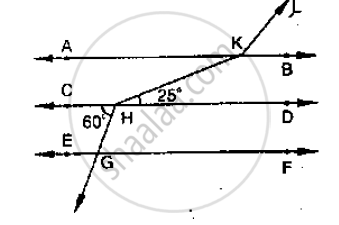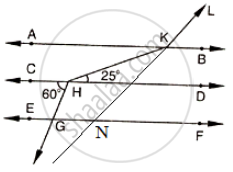# In the Below Fig, Ab || Cd || Ef and Gh || Kl. Find ∠ Hkl - Mathematics

Sum

In the below fig, AB || CD || EF and GH || KL. Find ∠HKL#### Solution

Produce LK to meet GF at N.Now, alternative angles are equal

∠CHG = ∠HGN = 60°

∠HGN = ∠KNF = 60°          [Corresponding angles]

∴∠KNG = 180° - 60° = 120°

∠GNK = ∠AKL = 120°                [Corresponding angles]

∠AKH = ∠KHD = 25°                [Alternative angles]

∴∠HKL = ∠AKH + ∠AKL = 25° +120° = 145°.

Concept: Parallel Lines and a Transversal
Is there an error in this question or solution?

#### APPEARS IN

RD Sharma Mathematics for Class 9
Chapter 10 Lines and Angles
Exercise 10.4 | Q 20 | Page 48